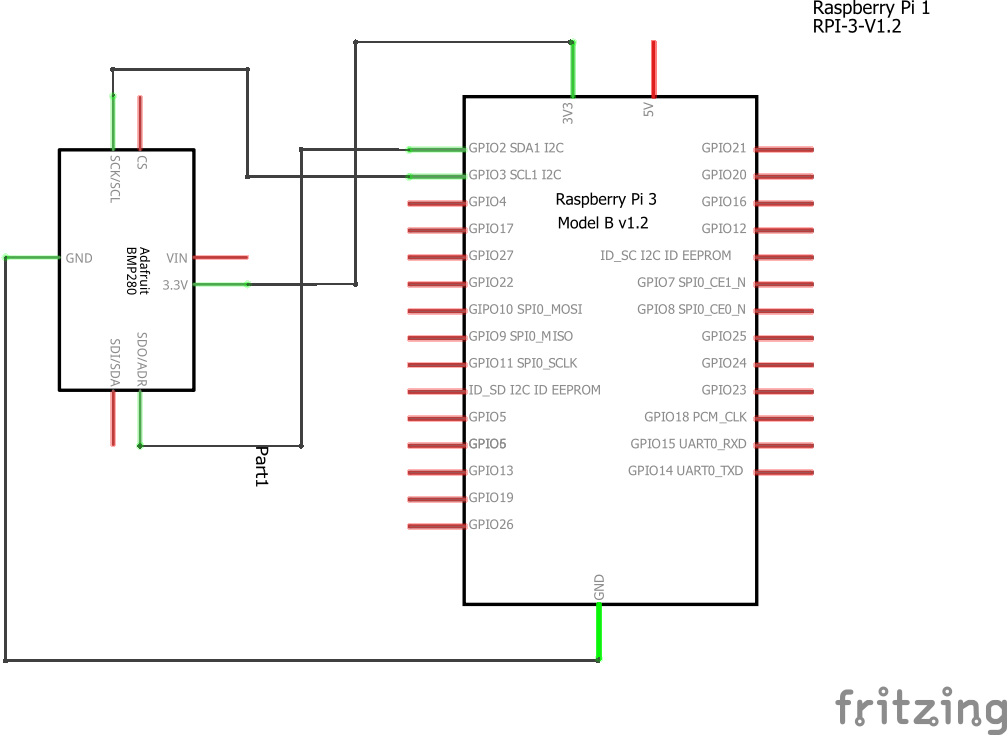Home Code Raspberry Pi and BMP280 sensor example

# Raspberry Pi and BMP280 sensor example

BMP280 is an absolute barometric pressure sensor especially designed for mobile applications. The sensor module is housed in an extremely compact package. Its small dimensions and its low power consumption allow for the implementation in battery powered devices such as mobile phones, GPS modules or watches.As its predecessor BMP180, BMP280 is based on Bosch’s proven Piezo-resistive pressure sensor technology featuring high accuracy and linearity as well as long term stability and high EMC robustness. Numerous device operation options offer highest flexibility to optimize the device regarding power consumption, resolution and filter performance. A tested set of default settings for example use case is provided to the developer in order to make design-in as easy as possible.

Applications

– Indoor navigation (floor detection, elevator detection)

– Outdoor navigation, leisure and sports applications

– Weather forecast

– Health care applications (e.g. spirometry)

– Vertical velocity indication (e.g. rise/sink speed)

Parameter Technical data
Operation range (full accuracy) Pressure: 300…1100 hPa
Temperature: -40…85°C
Absolute accuracy
(Temp. @ 0…+65°C)
~ ±1 hPa
Relative accuracy
p = 700…900hPa
(Temp. @ +25…+40°C)
± 0.12 hPa (typical)
equivalent to ±1 m
Average current consumption (1 Hz data refresh rate) 2.74 μA, typical
(ultra-low power mode)
Average current consumption in sleep mode 0.1 μA
Average measurement time 5.5 msec
(ultra-low power preset)
Supply voltage VDDIO 1.2 … 3.6 V
Supply voltage VDD 1.71 … 3.6 V
Resolution of data Pressure: 0.01 hPa ( < 10 cm)
Temperature: 0.01° C
Temperature coefficient offset
(+25°…+40°C @900hPa)
± 0.12 hPa (typical)
equivalent to ±1 m
Interface I²C and SPI

Layout

This is a layout diagram using an adafruit part, my module had clearly marked SDA and SCL connections

In this example we will connect to a raspberry PiCode

 Source code```# Distributed with a free-will license.
# Use it any way you want, profit or free, provided it fits in the licenses of its associated works.
# BMP280
# This code is designed to work with the BMP280_I2CS I2C Mini Module available from ControlEverything.com.
# https://www.controleverything.com/content/Barometer?sku=BMP280_I2CSs#tabs-0-product_tabset-2
import smbus
import time
# Get I2C bus
bus = smbus.SMBus(1)
# Read data back from 0x88(136), 24 bytes
# Convert the data
# Temp coefficents
dig_T1 = b1 * 256 + b1
dig_T2 = b1 * 256 + b1
if dig_T2 > 32767 :
dig_T2 -= 65536
dig_T3 = b1 * 256 + b1
if dig_T3 > 32767 :
dig_T3 -= 65536
# Pressure coefficents
dig_P1 = b1 * 256 + b1
dig_P2 = b1 * 256 + b1
if dig_P2 > 32767 :
dig_P2 -= 65536
dig_P3 = b1 * 256 + b1
if dig_P3 > 32767 :
dig_P3 -= 65536
dig_P4 = b1 * 256 + b1
if dig_P4 > 32767 :
dig_P4 -= 65536
dig_P5 = b1 * 256 + b1
if dig_P5 > 32767 :
dig_P5 -= 65536
dig_P6 = b1 * 256 + b1
if dig_P6 > 32767 :
dig_P6 -= 65536
dig_P7 = b1 * 256 + b1
if dig_P7 > 32767 :
dig_P7 -= 65536
dig_P8 = b1 * 256 + b1
if dig_P8 > 32767 :
dig_P8 -= 65536
dig_P9 = b1 * 256 + b1
if dig_P9 > 32767 :
dig_P9 -= 65536
# Select Control measurement register, 0xF4(244)
# 0x27(39) Pressure and Temperature Oversampling rate = 1
# Normal mode
bus.write_byte_data(0x76, 0xF4, 0x27)
# Select Configuration register, 0xF5(245)
# 0xA0(00) Stand_by time = 1000 ms
bus.write_byte_data(0x76, 0xF5, 0xA0)
time.sleep(0.5)
# Read data back from 0xF7(247), 8 bytes
# Pressure MSB, Pressure LSB, Pressure xLSB, Temperature MSB, Temperature LSB
# Temperature xLSB, Humidity MSB, Humidity LSB
# Convert pressure and temperature data to 19-bits
adc_p = ((data * 65536) + (data * 256) + (data & 0xF0)) / 16
adc_t = ((data * 65536) + (data * 256) + (data & 0xF0)) / 16
# Temperature offset calculations
var1 = ((adc_t) / 16384.0 - (dig_T1) / 1024.0) * (dig_T2)
var2 = (((adc_t) / 131072.0 - (dig_T1) / 8192.0) * ((adc_t)/131072.0 - (dig_T1)/8192.0)) * (dig_T3)
t_fine = (var1 + var2)
cTemp = (var1 + var2) / 5120.0
fTemp = cTemp * 1.8 + 32
# Pressure offset calculations
var1 = (t_fine / 2.0) - 64000.0
var2 = var1 * var1 * (dig_P6) / 32768.0
var2 = var2 + var1 * (dig_P5) * 2.0
var2 = (var2 / 4.0) + ((dig_P4) * 65536.0)
var1 = ((dig_P3) * var1 * var1 / 524288.0 + ( dig_P2) * var1) / 524288.0
var1 = (1.0 + var1 / 32768.0) * (dig_P1)
p = (p - (var2 / 4096.0)) * 6250.0 / var1
var1 = (dig_P9) * p * p / 2147483648.0
var2 = p * (dig_P8) / 32768.0
pressure = (p + (var1 + var2 + (dig_P7)) / 16.0) / 100
# Output data to screen
print "Temperature in Celsius : %.2f C" %cTemp
print "Temperature in Fahrenheit : %.2f F" %fTemp
print "Pressure : %.2f hPa " %pressure```

Output